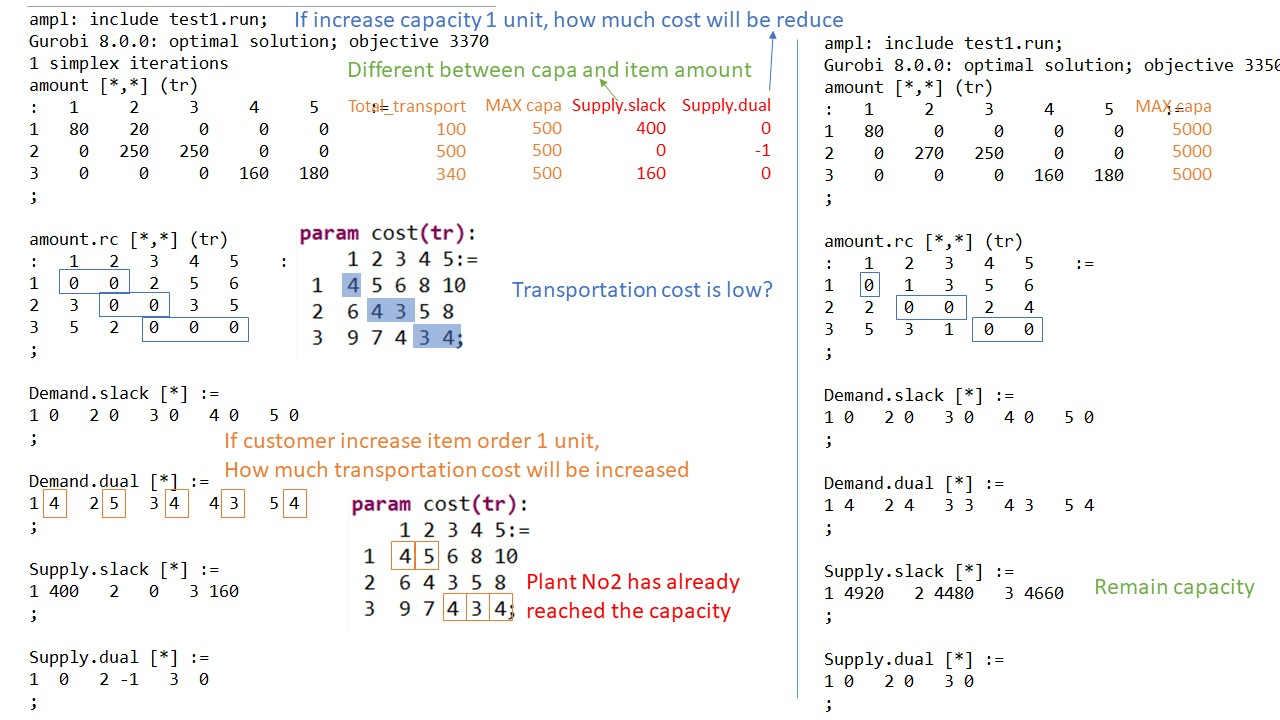## Duality

Transportation problem is defind as (Primal model)

• we have muktiple factory and multiple customer
• each factory has a capacity amount of items
• each customer place an order amount of items
• there are a different cost of sending 1 item from factoryABC to customerABCD
• To minimize transportation cost,
• which factory should send item to each customer
• and how many items do that factory send.

In another view of problem (Duality model – provide vital economic interpretations)

• If we want to expand a factory (to increase a capacity per factory), How much cost will be reduced.
• If customers order more items, How much profit we will get.

shadow price  :the optimal values of dual variables associate with each constraint

• is a price that paid for 1 unit of resource.

reduced cost : associate with each decision variable. defined as the change in objective function value

• is also called opportunity cost.## Mathematical Optimization

Mathematical Optimization = finding the best solution base on a given ojective function

Objective function = maximize or minimize

Linear optimization (a1x1+a2x2+…+anxn) : most basic

– Integeroptimization: more complicate (NP-class)
Ex. find maximum number of chicken and rabbit, while theare are 5 heads and 16 feet.

Non-linear optimization : difficult to solve

-Quadric optimization (x^2+xy) polinomial up to 2 : able to solve by using SCIP (especially if convex function)

To solve

1. define mathematical fomular

• variables :
• x1
• x2
• objective function :
• maximize 25×1+30×2
• contraints :
• 0<=x1<=6000
• 0<=x2<=4000
• x1/200+x2/140<=40

2. write  AMPL code from mathematical formular

var XB;
var XC;
maximize Profit: 25 * XB + 30 * XC;
subject to Time: (1/200) * XB + (1/140) * XC <= 40;
subject to B_limit: 0 <= XB <= 6000;
subject to C_limit: 0 <= XC <= 4000;